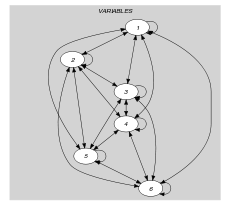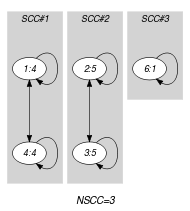## 5.289. nvalues_except_0

Origin
Constraint

$\mathrm{𝚗𝚟𝚊𝚕𝚞𝚎𝚜}_\mathrm{𝚎𝚡𝚌𝚎𝚙𝚝}_\mathtt{0}\left(\mathrm{𝚅𝙰𝚁𝙸𝙰𝙱𝙻𝙴𝚂},\mathrm{𝚁𝙴𝙻𝙾𝙿},\mathrm{𝙻𝙸𝙼𝙸𝚃}\right)$

Arguments
 $\mathrm{𝚅𝙰𝚁𝙸𝙰𝙱𝙻𝙴𝚂}$ $\mathrm{𝚌𝚘𝚕𝚕𝚎𝚌𝚝𝚒𝚘𝚗}\left(\mathrm{𝚟𝚊𝚛}-\mathrm{𝚍𝚟𝚊𝚛}\right)$ $\mathrm{𝚁𝙴𝙻𝙾𝙿}$ $\mathrm{𝚊𝚝𝚘𝚖}$ $\mathrm{𝙻𝙸𝙼𝙸𝚃}$ $\mathrm{𝚍𝚟𝚊𝚛}$
Restrictions
 $\mathrm{𝚛𝚎𝚚𝚞𝚒𝚛𝚎𝚍}$$\left(\mathrm{𝚅𝙰𝚁𝙸𝙰𝙱𝙻𝙴𝚂},\mathrm{𝚟𝚊𝚛}\right)$ $\mathrm{𝚁𝙴𝙻𝙾𝙿}\in \left[=,\ne ,<,\ge ,>,\le \right]$
Purpose

Let $N$ be the number of distinct values, different from 0, assigned to the variables of the $\mathrm{𝚅𝙰𝚁𝙸𝙰𝙱𝙻𝙴𝚂}$ collection. Enforce condition $N\mathrm{𝚁𝙴𝙻𝙾𝙿}\mathrm{𝙻𝙸𝙼𝙸𝚃}$ to hold.

Example
$\left(〈4,5,5,4,0,1〉,=,3\right)$

The $\mathrm{𝚗𝚟𝚊𝚕𝚞𝚎𝚜}_\mathrm{𝚎𝚡𝚌𝚎𝚙𝚝}_\mathtt{0}$ constraint holds since the number of distinct values, different from 0, occurring within the collection $〈4,5,5,4,0,1〉$ is equal (i.e., $\mathrm{𝚁𝙴𝙻𝙾𝙿}$ is set to $=$) to its third argument $\mathrm{𝙻𝙸𝙼𝙸𝚃}=3$.

Typical
 $|\mathrm{𝚅𝙰𝚁𝙸𝙰𝙱𝙻𝙴𝚂}|>1$ $\mathrm{𝙻𝙸𝙼𝙸𝚃}>1$ $\mathrm{𝙻𝙸𝙼𝙸𝚃}<|\mathrm{𝚅𝙰𝚁𝙸𝙰𝙱𝙻𝙴𝚂}|$ $\mathrm{𝚊𝚝𝚕𝚎𝚊𝚜𝚝}$$\left(1,\mathrm{𝚅𝙰𝚁𝙸𝙰𝙱𝙻𝙴𝚂},0\right)$ $\mathrm{𝚁𝙴𝙻𝙾𝙿}\in \left[=,<,\ge ,>,\le \right]$
Symmetries
• Items of $\mathrm{𝚅𝙰𝚁𝙸𝙰𝙱𝙻𝙴𝚂}$ are permutable.

• All occurrences of two distinct values of $\mathrm{𝚅𝙰𝚁𝙸𝙰𝙱𝙻𝙴𝚂}.\mathrm{𝚟𝚊𝚛}$ that are both different from 0 can be swapped; all occurrences of a value of $\mathrm{𝚅𝙰𝚁𝙸𝙰𝙱𝙻𝙴𝚂}.\mathrm{𝚟𝚊𝚛}$ that is different from 0 can be renamed to any unused value that is also different from 0.

Arg. properties
• Contractible wrt. $\mathrm{𝚅𝙰𝚁𝙸𝙰𝙱𝙻𝙴𝚂}$ when $\mathrm{𝚁𝙴𝙻𝙾𝙿}\in \left[<,\le \right]$.

• Extensible wrt. $\mathrm{𝚅𝙰𝚁𝙸𝙰𝙱𝙻𝙴𝚂}$ when $\mathrm{𝚁𝙴𝙻𝙾𝙿}\in \left[\ge ,>\right]$.

Reformulation

The $\mathrm{𝚗𝚟𝚊𝚕𝚞𝚎𝚜}_\mathrm{𝚎𝚡𝚌𝚎𝚙𝚝}_\mathtt{0}$$\left(〈{V}_{1},{V}_{2},\cdots ,{V}_{|\mathrm{𝚅𝙰𝚁𝙸𝙰𝙱𝙻𝙴𝚂}|}〉,\mathrm{𝚁𝙴𝙻𝙾𝙿},\mathrm{𝙻𝙸𝙼𝙸𝚃}\right)$ constraint can be expressed in term of the conjunction $\mathrm{𝚗𝚟𝚊𝚕𝚞𝚎}$$\left(\mathrm{𝑁𝑉}\mathit{1},〈0,{V}_{1},{V}_{2},\cdots ,{V}_{|\mathrm{𝚅𝙰𝚁𝙸𝙰𝙱𝙻𝙴𝚂}|}〉\right)$ $\wedge$ $\mathrm{𝑁𝑉}\mathit{1}-1\mathrm{𝚁𝙴𝙻𝙾𝙿}\mathrm{𝙻𝙸𝙼𝙸𝚃}$.

Used in
Keywords
Arc input(s)

$\mathrm{𝚅𝙰𝚁𝙸𝙰𝙱𝙻𝙴𝚂}$

Arc generator
$\mathrm{𝐶𝐿𝐼𝑄𝑈𝐸}$$↦\mathrm{𝚌𝚘𝚕𝚕𝚎𝚌𝚝𝚒𝚘𝚗}\left(\mathrm{𝚟𝚊𝚛𝚒𝚊𝚋𝚕𝚎𝚜}\mathtt{1},\mathrm{𝚟𝚊𝚛𝚒𝚊𝚋𝚕𝚎𝚜}\mathtt{2}\right)$

Arc arity
Arc constraint(s)
 $•\mathrm{𝚟𝚊𝚛𝚒𝚊𝚋𝚕𝚎𝚜}\mathtt{1}.\mathrm{𝚟𝚊𝚛}\ne 0$ $•\mathrm{𝚟𝚊𝚛𝚒𝚊𝚋𝚕𝚎𝚜}\mathtt{1}.\mathrm{𝚟𝚊𝚛}=\mathrm{𝚟𝚊𝚛𝚒𝚊𝚋𝚕𝚎𝚜}\mathtt{2}.\mathrm{𝚟𝚊𝚛}$
Graph property(ies)
$\mathrm{𝐍𝐒𝐂𝐂}$$\mathrm{𝚁𝙴𝙻𝙾𝙿}\mathrm{𝙻𝙸𝙼𝙸𝚃}$

Graph model

Parts (A) and (B) of Figure 5.289.1 respectively show the initial and final graph associated with the Example slot. Since we use the $\mathrm{𝐍𝐒𝐂𝐂}$ graph property we show the different strongly connected components of the final graph. Each strongly connected component corresponds to a value distinct from 0 that is assigned to some variables of the $\mathrm{𝚅𝙰𝚁𝙸𝙰𝙱𝙻𝙴𝚂}$ collection. Beside value 0, the 3 following values 1, 4 and 5 are assigned to the variables of the $\mathrm{𝚅𝙰𝚁𝙸𝙰𝙱𝙻𝙴𝚂}$ collection.

##### Figure 5.289.1. Initial and final graph of the $\mathrm{𝚗𝚟𝚊𝚕𝚞𝚎𝚜}_\mathrm{𝚎𝚡𝚌𝚎𝚙𝚝}_\mathtt{0}$ constraint(a) (b)